GMAT Math : Arithmetic

Example Questions

Example Question #3167 : Gmat Quantitative Reasoning

Two dice are thrown; one is fair, one is loaded, but each has the usual numbers 1-6 on its faces. What is the probability of the outcome being a sum of 12?

Statement 1: The loaded die will come up 6 with probability.

Statement 2: The loaded die will come up 5 with probability.

Statement 2 ALONE is sufficient to answer the question, but Statement 1 ALONE is NOT sufficient to answer the question.

EITHER statement ALONE is sufficient to answer the question.

Statement 1 ALONE is sufficient to answer the question, but Statement 2 ALONE is NOT sufficient to answer the question.

BOTH statements TOGETHER are sufficient to answer the question, but NEITHER statement ALONE is sufficient to answer the question.

BOTH statements TOGETHER are insufficient to answer the question.

Statement 1 ALONE is sufficient to answer the question, but Statement 2 ALONE is NOT sufficient to answer the question.

Explanation:

The only way the sum of the two dice can come up 12 is a pair of sixes, so the only probabilities that count are those of each die coming up 6; Statement 2 is therefore irrelevant to the problem. If you are given Statement 1, however, then you can calculate the probability of tossing a 12 by multiplying: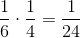Example Question #3168 : Gmat Quantitative Reasoning

One hundred marbles, 75 red and 25 white, are distributed equally between two boxes, denoted Box 1 and Box 2. Box 1 contains 30 red marbles.

One box is chosen at random, and one marble is chosen at random from that box. What is the probability that the marble chosen will be white?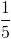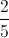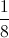Explanation:

Box 1 will contain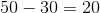white marbles; Box 2 will contain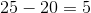white marbles.

The probability of choosing Box 1 is, and the probability of choosing a white marble from this box is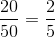, making the probability of choosing Box 1, then a white marble,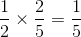.

The probability of choosing Box 2 is, and the probability of choosing a white marble from this box is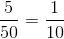, making the probability of choosing Box 2, then a white marble,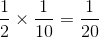.

The overall probability of choosing a white marble is the sum of these probabilities: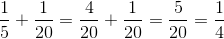Example Question #3169 : Gmat Quantitative Reasoning

You are given two boxes, each with an equal number of marbles. All of the marbles are red or white. Are you more likely to draw a red marble at random from Box 1 or from Box 2?

Statement 1: Two-thirds of all the marbles are red.

Statement 2: Half the marbles in Box 2 are red.

BOTH statements TOGETHER are insufficient to answer the question.

BOTH statements TOGETHER are sufficient to answer the question, but NEITHER statement ALONE is sufficient to answer the question.

Statement 2 ALONE is sufficient to answer the question, but Statement 1 ALONE is NOT sufficient to answer the question.

EITHER statement ALONE is sufficient to answer the question.

Statement 1 ALONE is sufficient to answer the question, but Statement 2 ALONE is NOT sufficient to answer the question.

BOTH statements TOGETHER are sufficient to answer the question, but NEITHER statement ALONE is sufficient to answer the question.

Explanation:

Since both boxes contain the same number of marbles, the one with the most red marbles offers us the greater probability of drawing a red marble. For simplicity's sake, assume that each box contains thirty marbles - these arguments will be valid whatever the number.

From Statement 1, it follows that two-thirds of sixty, or forty, marbles total are red, but either box can contain more than the other.

From Statement 2, it follows that fifteen marbles in Box 2 are red, but no information about Box 1 follows.

From both statements, however, it follows that there are fifteen red marbles in Box 2 and twenty-five red marbles in Box 1, so Box 1 has more red marbles and offers the greater chance of drawing a red marble.

Example Question #3170 : Gmat Quantitative Reasoning

You are given two boxes of marbles, all either red or white. Are you more likely to draw a red marble at random from Box 1 or from Box 2?

Statement 1: Each box contains the same number of red marbles.

Statement 2: Box 2 contains twice as many white marbles as Box 1.

EITHER statement ALONE is sufficient to answer the question.

Statement 1 ALONE is sufficient to answer the question, but Statement 2 ALONE is NOT sufficient to answer the question.

BOTH statements TOGETHER are insufficient to answer the question.

BOTH statements TOGETHER are sufficient to answer the question, but NEITHER statement ALONE is sufficient to answer the question.

Statement 2 ALONE is sufficient to answer the question, but Statement 1 ALONE is NOT sufficient to answer the question.

BOTH statements TOGETHER are sufficient to answer the question, but NEITHER statement ALONE is sufficient to answer the question.

Explanation:

If only Statement 1 is assumed, there is no way of knowing which box offers the highest probability of drawing a red marble, since there is no way to tell which box has the higher portion of its marbles red without knowing how their numbers of white marbles or their total numbers of marbles compare. A smiliar argument holds for Statement 2 alone.

If both statements are known, however, each box has the same number of red marbles, and Box 2 contains more marbles overall - this makes Box 1 the box with the highest portion of its marbles red, and the box that offers the greater chance of drawing a red marble in a random draw.

Example Question #21 : Dsq: Calculating Discrete Probability

Two dice are thrown and their sum is noted. One is fair and one is loaded but both have the usual numbers 1-6 on their faces. Which, if either, is greater - the probability of tossing a 3 or the probability of tossing an 11?

Statement 1: The probability of tossing a 2 on the loaded die is 0.2.

Statement 2: The probability of tossing a 5 on the loaded die is 0.3.

EITHER statement ALONE is sufficient to answer the question.

Statement 2 ALONE is sufficient to answer the question, but Statement 1 ALONE is NOT sufficient to answer the question.

BOTH statements TOGETHER are sufficient to answer the question, but NEITHER statement ALONE is sufficient to answer the question.

BOTH statements TOGETHER are insufficient to answer the question.

Statement 1 ALONE is sufficient to answer the question, but Statement 2 ALONE is NOT sufficient to answer the question.

BOTH statements TOGETHER are insufficient to answer the question.

Explanation:

A 3 can be rolled only by tossing a 1-2, but the loaded die can be either the 1 or the 2; similarly, an 11 can be rolled only by tossing a 5-6, but the loaded die can be either the 5 or the 6. We know only the probability of the loaded die coming up 2 and that of it coming up 5; we do not have this information for 1 or 6. We explore two cases and compare the probabilities of rolling a 3 and rolling an 11.

Case 1: Suppose the loaded die will come up 1 with probability 0.1 and 6 with probability 0.3. The probabilities of rolling a 3 or a 11 are, respectively: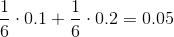and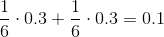making 11 the more likely outcome.

Case 2: Suppose the loaded die will come up 1 with probability 0.3 and 6 with probability 0.1. The probabilities of rolling a 3 or a 11 are, respectively: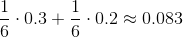and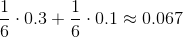making 3 the more likely outcome.

Therefore, the two statements together provide insufficient information to answer the question.

Example Question #3172 : Gmat Quantitative Reasoning

Some balls - some completely red, some completely yellow, the rest half red and half yellow - are placed in a large box, and a ball is drawn at random. Which probability is greater - the probability of drawing a ball that is at least partly red, or the probability of drawing a ball that is at least partly yellow?

Statement 1: The ratio of balls that are half red and half yellow to balls that are completely red is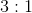.

Statement 2: The ratio of completely yellow balls to completely red balls is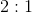Statement 1 ALONE is sufficient to answer the question, but Statement 2 ALONE is NOT sufficient to answer the question.

BOTH statements TOGETHER are insufficient to answer the question.

EITHER statement ALONE is sufficient to answer the question.

BOTH statements TOGETHER are sufficient to answer the question, but NEITHER statement ALONE is sufficient to answer the question.

Statement 2 ALONE is sufficient to answer the question, but Statement 1 ALONE is NOT sufficient to answer the question.

Statement 2 ALONE is sufficient to answer the question, but Statement 1 ALONE is NOT sufficient to answer the question.

Explanation:

Let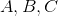be the balls that are completely red, completely yellow, and bicolored, respectively; let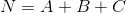be the total number of balls. Then the probabilities of drawing a ball with some red and drawing a ball with some yellow are, respectively,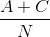and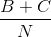For the former probability to be greater than the latter, we can use some algebra to reveal: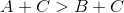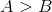That is, the former probability is greater than the latter if and only if there are more completely red balls than yellow balls. Similarly, the former probability is less than the latter if and only if there are fewer completely red balls than yellow balls.

Therefore, Statement 1 is irrelevant; Statement 2, which compares the number of completely red balls and completely yellow balls, gives us our necessary and sufficient information.

Example Question #3173 : Gmat Quantitative Reasoning

Two fair dice are tossed. What is the probability that the difference of the two numbers will be 1?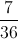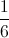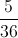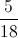Explanation:

The difference of the two dice will be 1 in case any of the following outcomes occur: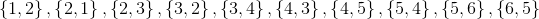This is ten outcomes out of a possible 36, so this yields a probability of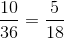.

Example Question #3174 : Gmat Quantitative Reasoning

For Data Sufficiency questions, select either A, B, C, D, E based on the following rubric:

• A) statement 1 alone is sufficient, but statement 2 alone is not sufficient to answer the question
• (B) statement 2 alone is sufficient, but statement 1 alone is not sufficient to answer the question
• (C) both statements taken together are sufficient to answer the question, but neither statement alone is sufficient
• (D) each statement alone is sufficient
• (E) statements 1 and 2 together are not sufficient, and additional data is needed to answer the question

When a delectable baked treat is selected at random from the baker's case, what is the probability that it will be kirsch flavored?

1. One half of the treats are peanut-butter flavored.

2. There are one third as many kirsch treats as peanut-butter treats.

C

D

E

A

B

C

Explanation:

Remember, you do not have to SOLVE the data sufficiency problems.  In other words, you do not have to calculate the exact probability of getting a kirsch-flavored (cherry liquor) treat.  You just need to determine how many of the statements are NECESSARY to derive the answer.

The first statement by itself is not sufficient, because knowing that half of the treats are peanut butter flavored does not tell you the proportion that are kirsch flavored.

The second statement is not sufficient, because comparing the proportion of kirsch treats to peanut-butter treats won't help you find the probability of getting a kirsch treat unless you know the ratio of peanut-butter treats in the case.

But, if we put the two pieces of information together, we can solve the problem.  Statement 1 tells us the proportion of peanut-butter treats, and statement 2 tells us how many kirsch treats there are compared to peanut-butter treats.

Therefore, the correct answer is C.

Example Question #3175 : Gmat Quantitative Reasoning

Anna is about to pick a fruit for her snack from a basket of apples and oranges. What is the probability Anna picks an apple?

(1) The basket containsfruits.

(2) Half of the fruits in the basket are oranges.

D: EACH statement ALONE is sufficient

E: Statements (1) and (2) TOGETHER are not sufficient

A: Statement (1) ALONE is sufficient, but statement (2) ALONE is not sufficient

B: Statement (2) ALONE is sufficient, but statement (1) ALONE is not sufficient

C: BOTH statements TOGETHER are sufficient, but NEITHER statement ALONE is sufficient

B: Statement (2) ALONE is sufficient, but statement (1) ALONE is not sufficient

Explanation:

Let P(A) be the probability of Anna picking an apple and P(O) be the probability of Anna picking an orange. We need to evaluate each statement individually first:

(1) gives us the total number of fruits, but no indication as to the number of each fruit, so we cannot determine probabilities form (1) alone

(2) gives us the proportion of oranges. Let N be the total number of fruits, A the number of apples and O the number of oranges. We have: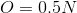We also know that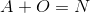.

We can rewrite this last equation as follow: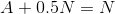or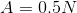We know that P(A) = A / N and from statement (2) we get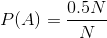which we can simplify to find P(A)

Therefore the right answer is B.

Example Question #3176 : Gmat Quantitative Reasoning

What is the probability that a randomly selected person in a sample of 100 adults is left handed?

(1) The number of left handed adults in the sample represents one fourth of the number of right handed adults in the sample.

(2) All of the women in the sample are right-handed.

Both statements TOGETHER are sufficient, but NEITHER statement ALONE is sufficient

Both statements TOGETHER are not sufficient.

Statement (1) ALONE is sufficient, but Statement (2) ALONE is not sufficient

Each statement ALONE is sufficient.

Statement (2) ALONE is sufficient, but Statement (1) ALONE is not sufficient

Statement (1) ALONE is sufficient, but Statement (2) ALONE is not sufficient

Explanation:

Using Statement (1), we can write the following equation:

Let x be the number of left handed people in the sample. 100-x is then the number of right handed people in the sample.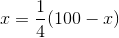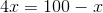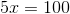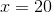Therefore, the probability that a randomly selected person in the sample is left handed is: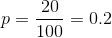Therefore Statement (1) is sufficient to answer the question

Statement (2) indicates that all women in the sample are right handed. This statement is not useful as it does not give the total number of right handed people in the sample.

Therefore, Statement (2) is not sufficient by itself to answer the question.

Only Statement (1) ALONE is sufficient.

Tired of practice problems?

Try live online GMAT prep today.